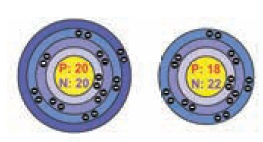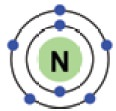Home | | Science 9th std | Isotopes (Iso ŌĆō same, topo ŌĆō place, Isotope ŌĆō same place

# Isotopes (Iso ŌĆō same, topo ŌĆō place, Isotope ŌĆō same place

All the three structures have same atomic number but different mass numbers.

Isotopes (Iso ŌĆō same, topo ŌĆō place, Isotope ŌĆō  same place

## 1. ISOTOPES

Find below three different atoms. Count the different subatomic particles and fill in the table.### Which is same and what is different in them?

All of them have the same number of protons and electrons but different number of neutrons.

### What will they have in common?

All the three structures have same atomic number but different mass numbers. They have the same number of electrons also. Such atoms of the same element are called isotopes.

Isotopes are atoms of the same element having same atomic number but different mass numbers.

This is due to the difference in the number of neutrons in the nucleus. Isotopes differ in few physical properties such as density, boiling point etc. Physical properties depend upon mass number. Isotopes have different mass numbers. So they differ in physical properties.Example: Isotopes of carbon

### Why do some isotopes show radioactivity?

When the number of neutrons exceeds the number of protons in the nucleus of atoms, some nuclei become unstable. These unstable nuclei break up spontaneously emitting certain type of radiations. They are known as radioactive isotopes. Examples: H3and C14

Many elements have isotopes of which some of them are radioactive isotopes.

Radioactivity prevails around us. The food we eat, the air we breathe, the buildings we live in, all contain small amounts of radioactive materials. This radiation will be present always.

Thus there are a lot of low level natural radioactivity around us. For example, our bodies contain radioisotopes, such as potassium-40, which continuously emit radiation, but the level is so low that this does not harm us.The picture below shows us how radioactive carbon(C14) is all around us.But the special properties of radioactive isotopes make them useful to us in various fields.## 2. Isobars### What is the difference between these two atoms?

The above two elements calcium and argon have atomic number 20 and atomic number 18 respectively. is means they have different number of protons and electrons. But the mass number of both these elements is 40. It follows that the total number of nucleons in both of them are the same. Atoms of different elements with different atomic numbers, which have the same mass number, are known as isobars.

## 3. IsotonesThe above pair of elements Boron and Carbon has the same number of neutrons but different number of protons and hence different atomic numbers. Atoms of different elements with different atomic numbers and different mass numbers, but with the same number of neutrons are called isotones.

### How are electrons arranged around the nucleus in an atom?

So far we have been discussing about the nucleus of an atom and the protons and neutrons which constitute the nucleus. We also saw that electrons are extra nuclear particles and they revolve around the nucleus in fixed trajectories or orbits. Let us now see how electrons are arranged in different orbits. The systematic arrangement of electrons in various shells or orbits in an atom is called the electronic configuration.

### Electronic configuration of atoms:

You already know that electrons occupy different energy levels called orbits or shells.The distribution of electrons in these orbits of an atom is governed by certain rules or conditions. These are known as Bohr and Bury Rules of electronic configuration.

Bohr and Bury simultaneously proposed the following rules for the distribution of electrons in different shells.

┬Ę        Rule 1: The maximum number of electrons that can be accommodated in a shell is equal to 2n2 where ŌĆśnŌĆÖ is the quantum number of the shell (i.e., the serial number of the shell from the nucleus).┬Ę        Rule 2: Shells are filled in a stepwise manner in the increasing order of energy.

┬Ę        Rule 3: The outermost shell cannot have more than 8 electrons and the next inner, i.e., the penultimate shell cannot have more than 18 electrons.

Illustration:

Structure of Aluminium atom: (13 electrons) K shell = 2 electron, L shell = 8, M Shell ŌĆō 3

So its electronic con guration is 2, 8, 3### Electronic configuration of first 20 elementsFor getting a basic idea about the electron distribution around the nucleus we can draw schematic diagrams as shown below. As you learn more about atomic structure you will come to know that the real picture of electron distribution is entirely different from what we have shown here.

Schematic diagrams for Atomic Structure of Elements (first 20)## 4. Valence electrons

How many electrons are in the outermost shell? 5The outermost shell of an atom is called its valence shell and the electrons present in the valence shell are known as valence electrons.Hydrogen atom has only one electron in its valence shell. Hence it has one valence electron. Similarly carbon has 4 electrons in the outermost shell and so it has 4 valence electrons.

The chemical properties of elements are decided by these valence electrons, since they are the ones that take part in chemical reaction.

The elements with same number of electrons in the valence shell show similar properties and those with different number of valence electrons show different chemical properties. Elements, which have valence electrons 1 or 2 or 3 (except Hydrogen) are metals.

These elements can lose electrons to form ions which are positively charged and are called cations.Elements with 4 to 7 electrons in their valence shells are non-metals.

These elements can gain electrons to form ions which are negatively charged and are called anions.## 5. Valency

Valency of an element is the combining capacity of the element with other elements and is equal to the number of electrons that take part in a chemical reaction. Valency of the elements having valence electrons 1, 2, 3, 4 is 1, 2, 3, 4 respectively.

While valency of an element with 5, 6 &7 valence electrons is 3, 2 and 1 (8ŌĆōvalence electrons) respectively, where 8 is the number of electrons required by an element to attain stable electronic con guration. Elements having completely lled outermost shell show Zero valency.

For example: e electronic con guration of Neon is 2,8 (completely lled). So valency is 0.

Illustration:

Assign the valency of Magnesium & Sulphur

Electronic configuration of magnesium is 2, 8, 2. So valency is 2.

Electronic configuration of sulphur is 2, 8, 6. So valency is 2 i.e.(8 ŌĆō 6)

### Introduction to Quantum Numbers

We have learnt to designate orbits (shells) by K,L,M, N ŌĆ”., and orbitals (sub shells) as s, p, d and f. We have seen that electrons are lled in to these orbitals according to certain rules. Can we now designate an electron in an atom in a manner in which it gets a unique identity? Each electron inside of an atom has its own ŌĆśidentityŌĆÖ which is given by four quantum numbers that communicate a great deal of information about that electron.

The numbers which designate and distinguish various atomic orbitals and electrons present in an atom are called quantum numbers.

How would you describe to someone exactly where you live? I guess you would start with your address. (similar to the identity fo an electron).

When you specify the location of a building, you usually list which country it is in, which state and city it is in that country, then the area and the street and the door number.

Just like no two buildings have the exact same address, no two electrons can have the same set of quantum numbers.

A quantum number describes a speci c aspect of an electron. Just like we have four ways of de ning the location of a building (country, state, city, and street address), we have four ways of de ning the properties of an electron, i.e.four quantum numbers.

### These quantum numbers tell us

┬Ę        how far is the electron from the nucleus,-(Principal Quantum number)

┬Ę        which orbital does it occupy and what is its shape (Azimuthal Quantum number)

┬Ę        how this orbital is oriented in space (Magnetic Quantum number)

┬Ę        what kind of spin the electron has. (Spin Quantum number):